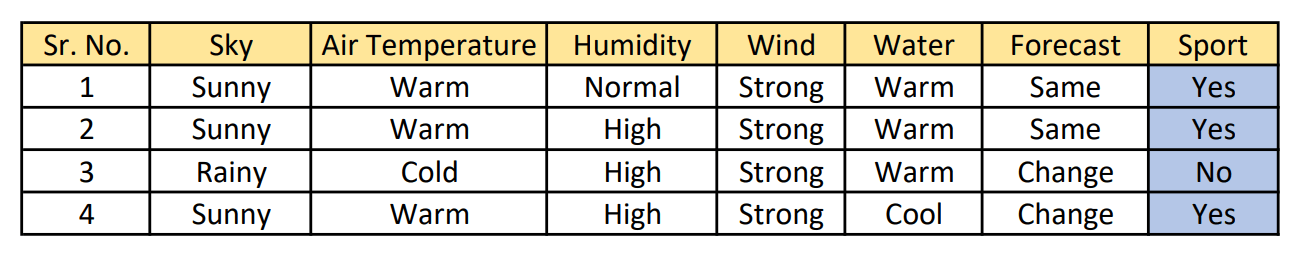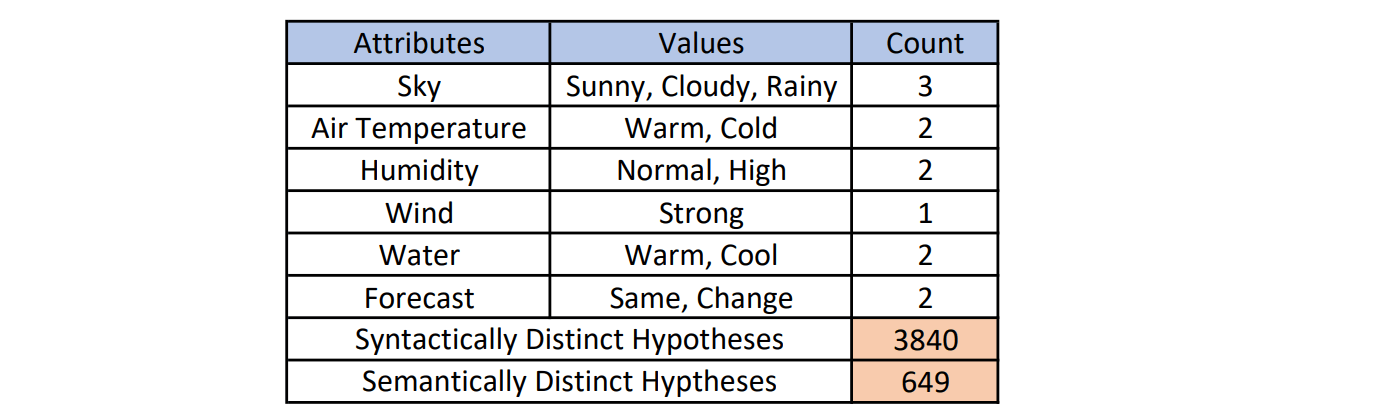## Introduction

To understand how a machine can learn from past experiences and predict the future based on the
experience gained, we need to understand the working of a human brain first. Once we find out
the pattern of a human brain to solve a problem, we can make our machine learn in almost the same way.

#### What is Learning?

Learning can be defined as the activity or process of gaining knowledge or skill by studying, practicing, being taught, or experiencing something. There are various categories of learning methods that are used by individuals in order to learn concepts and form an understanding. These learning methods are:

• Rote Learning: This method of learning is also called memorization, wherein we complete the agenda of learning without knowing the concept or logic behind them.
• Passive Learning: When we learn from teachers or experts by following the instructions given.
• Experiential Learning: Learning new things from our exact past experience.
• Inductive Learning: On the basis of past experience formulating a generalized concept by using analogies.
• Deductive Learning: Deriving new facts from past facts and learning concepts.

In the field of Machine Learning, the main focus is on inductive learning, where the formulation of a generalized concept-based rule after observing a number of instances of examples of the concept is the prime purpose. This simply means that we can make our machine learn things from past data and make them intelligent to identify whether an object falls into a specific category of our interest or not.

#### What is a Concept?

Let us assume that we have collected data for some attributes or features of the day like, Sky, Air, Temperature, Humidity, Wind, Water, and Forecast. Let these set of instances be denoted by X and many concepts can be defined over the X. For example, the concepts can be as follows:

• Whether a person can play their favorite water sport on a particular day.
• Whether a person will not be able to go outside their home on a particular day.
• Whether a person can enjoy on the beach on a particular day.

The answer to these questions can either be yes or no. Therefore, we can say that a concept is a boolean-valued function defined over a large set of objects or events.

#### What is Concept Learning?

From all that we have understood above, it can be implied that Concept Learning is defined as inferring a boolean-valued function from training examples of input and output of the function.

In Concept Learning, we aim to use data to teach a machine to solve a binary classification problem, i.e., to classify a data point as either belonging to or not belonging to a particular concept or idea.

## Concept Learning

Let us continue with the example above to understand about target concept (a concept or a function that is to be learned), denoted by c. Target concept can be seen as a boolean-valued function defined over X and can be represented as c: X→ {0, 1}. Here, X denotes the set of items or objects over which the concept is defined, usually called the set of instances.

For the target concept: “Whether a person can play their favorite water sport on a particular day”, an attribute ‘Sport’ is included in the below dataset X and it indicates whether or not a particular individual enjoys his favorite water sport on a day with the given conditions.Now the target concept is Sport: X →{0,1}. It is the mapping function f: X->y and this represents the exact knowledge defining the relationship between the set of input variables X and the output variable y. This target concept is unknown. With this data, a learner’s task is to learn to predict the value of Sport for any arbitrary day, based on the values of its attribute values. When a new sample with the values for attributes <Sky, Air, Temperature, Humidity, Wind, Water, Forecast> is given, the value for Sport (i.e., 0 or 1/No or Yes) is predicted based on the previous learning. Concepts can be learned using various algorithms that will be discussed in the forthcoming articles.

#### Hypothesis

A hypothesis is a proposition or a statement made on the basis of limited evidence as a starting point for further investigation. i.e., it is a function that best describes the target concept. The representation of a hypothesis is very simple. Each hypothesis is represented as a conjunction of constraints on the instance attributes. For this example, each hypothesis is a vector of six constraints, specifying the values of the six attributes <Sky, Air, Temperature, Humidity, Wind, Water, Forecast>. In hypothesis representation, the value of each attribute could be either one of the following:

• “?”: The question mark symbol represents that any value is acceptable for this attribute.
• Specific Label: A particular value is specified (e.g., Warm for the attribute Water).
• “ϕ”: The phi symbol represents that no value is acceptable.

Let us say that the hypothesis that an individual plays their favorite water sport only on cold days with high humidity only, and is independent of the values of the other attributes. This scenario can be represented by the expression:

< ?, Cold, High, ?, ? ,?>

The most general hypothesis indicates that no matter what conditions, the result will be always positive. This can be represented as:

< ?, ? , ? , ?, ? , ?>

The most specific hypothesis indicates that no day will we get a positive outcome to play the particular water sport. This can be represented by:

< ϕ, ϕ, ϕ, ϕ, ϕ, ϕ,>

When learning the target concept, the learner is presented with a set of training examples (x, c(x)), each consisting of an instance x from X, along with its target concept value c(x).

For positive examples, instances have c(x) = 1 and for negative examples, instances have c(x) = 0 . We will often write the ordered pair (x, c(x)) to describe the training example consisting of the instance x and its target concept value c(x).

The symbol “H” denotes the set of all possible hypotheses that the learner may consider regarding the identity of the target concept. So, H = {h1, h2, …. }.

The goal of the inductive learning algorithms in Concept Learning is to induce a “general rule” from a set of observed instances i.e., to find a hypothesis ‘h’ in H such that h(x)=c(x), for all x in X. But in reality, for a given set of examples, the learning algorithm returns a function h(x) that only approximates c.

Given a set of training examples of the target concept c, the task of the learner is to hypothesize or estimate c. Any hypothesis found to approximate the target function well over a sufficiently large set of training examples will also approximate the target function well over other unobserved examples.

#### Hypothesis Space

Let X denote the instances and H as hypotheses in the ‘Sport’ learning task. Each of the 6 attributes <Sky, Air Temperature, Humidity, Wind, Water, Forecast> can have the following distinct values:

• ‘Sky’ can have 3 possible values (sunny, cloudy, and rainy)
• ‘Air Temperature’ can have 2 possible values (warm and cloud)
• ‘Humidity’ can have 2 possible values (normal and high)
• ‘Wind’ has only 1 value (strong)
• ‘Water’ can have 2 possible values (warm and cool)
• ‘Forecast’ can have 2 possible values (same and change)

Hence, the total number of distinct instances is given as 3*2*2*1*2*2 = 48.
In the hypothesis, the value of each attribute could be either “?” or “ϕ” in addition to the other defined values. So, the total number of syntactically distinct hypotheses (hypothesis space) H is given as 5*4*4*3*4*4 = 3840

These 3840 syntactically distinct hypotheses are not semantically distinct. For example, the below 2 hypothesis says the same but they look different:

h1 = <Sky=ϕ AND Temp=warm AND Humidity=? AND Wind=strong AND Water=warm AND Forecast=same>

h2 = <Sky=sunny AND Temp=warm AND Humidity=? AND Wind=strong AND Water=ϕ AND Forecast=same>

Neither of these hypotheses accepts any day for Sport, so they are semantically the same. All such hypotheses having the same semantic is counted as 1. So, the total number of semantically distinct hypotheses is 1 (hypothesis with one or more ϕ) + 4×3×3×2×3×3 (add ? to each attribute) = 649 semantically
distinct hypotheses.So, concept learning can be viewed as the task of searching through a large space of hypotheses, to find the hypothesis that best fits the training examples.

A hypothesis “h” is consistent with a set of training examples D of target concept c if and only if h(x) = c(x) for each training example in D.

Let: h1 = <sunny, ?, ?, strong, ?, ?> and h2 = <sunny, ?, ?, ?, ?, ?>.

By looking at the 2 hypothesis representations, h2 has fewer constraints on attributes than h1. The sets of instances that are classified positive by h1 will be less than the sets of instances that are classified positive by h2. In fact, any instance classified positive by h1 will also be classified positive by h2. Therefore, we say that h2 is more general than h1 or h1 is more specific than h2.

Let:

x1 = <sunny, warm, high, strong, cool, same>

x2 = <sunny, cold, high, strong, warm, change>

h1 = <sunny, ?, ?, ?, ?, same>
h2 = <sunny, ?, ?, ?, ?, ?>
h3 = <sunny, ?, ?, ?, cool, ?>

Here, h1 classifies x1, h2 classifies x1 and x2 and h3 classifies x1. This indicates h2 is more general than h1 and h3. By taking advantage of the naturally occurring structure over the hypothesis space, we can design learning algorithms that can exhaustively search even infinite hypothesis spaces without explicitly enumerating every hypothesis.

We will see a few algorithms in the forthcoming articles.